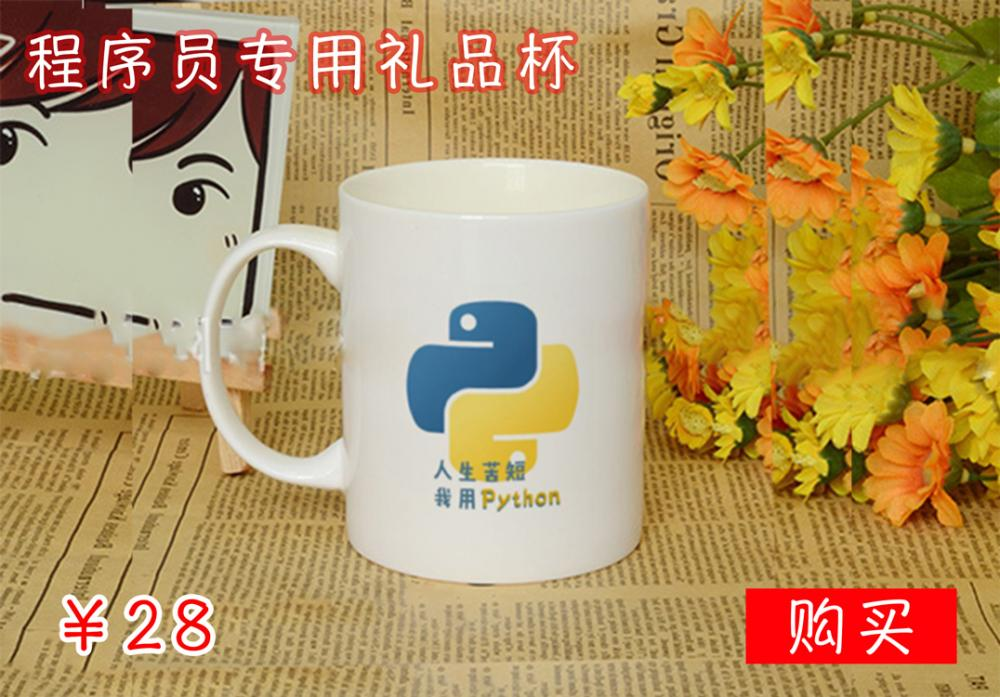1）忘记在 if , elif , else , for , while , class ,def 声明末尾添加 ：（导致 “SyntaxError ：invalid syntax”）

```if spam == 42
print('Hello!')```

2)使用 = 而不是 ==（导致“SyntaxError: invalid syntax”）

= 是赋值操作符而 == 是等于比较操作。该错误发生在如下代码中：

```if spam = 42:
print('Hello!')```

3）错误的使用缩进量。（导致“IndentationError：unexpected indent”、“IndentationError：unindent does not match any outer indetation level”以及“IndentationError：expected an indented block”）

```print('Hello!')
print('Howdy!')

if spam == 42:
print('Hello!')
print('Howdy!')

if spam == 42:
print('Hello!')```

4）for 循环语句中忘记调用 len() (导致“TypeError: ‘list’ object cannot be interpreted as an integer”)

```spam = ['cat', 'dog', 'mouse']
for i in range(spam):
print(spam[i])```

5）尝试修改string的值（导致“TypeError: ‘str’ object does not support item assignment”）

string是一种不可变的数据类型，该错误发生在如下代码中：

```spam = 'I have a pet cat.'
spam = 'r'
print(spam)```

```spam = 'I have a pet cat.'
spam = spam[:13] + 'r' + spam[14:]
print(spam)```

6）尝试连接非字符串值与字符串（导致 “TypeError: Can’t convert ‘int’ object to str implicitly”）

```numEggs = 12
print('I have ' + numEggs + ' eggs.')```

```numEggs = 12
print('I have ' + str(numEggs) + ' eggs.')

numEggs = 12
print('I have %s eggs.' % (numEggs))```

7）在字符串首尾忘记加引号（导致“SyntaxError: EOL while scanning string literal”）

```print(Hello!')

print('Hello!)

myName = 'Al'
print('My name is ' + myName + . How are you?')```

8）变量或者函数名拼写错误（导致“NameError: name ‘fooba’ is not defined”）

```foobar = 'Al'
print('My name is ' + fooba)

spam = ruond(4.2)

spam = Round(4.2)```

9）方法名拼写错误（导致 “AttributeError: ‘str’ object has no attribute ‘lowerr‘”）

```spam = 'THIS IS IN LOWERCASE.'
spam = spam.lowerr()```

10）引用超过list最大索引（导致“IndexError: list index out of range”）

```spam = ['cat', 'dog', 'mouse']
print(spam)```

11）使用不存在的字典键值（导致“KeyError：‘spam’”）

```spam = {'cat': 'Zophie', 'dog': 'Basil', 'mouse': 'Whiskers'}
print('The name of my pet zebra is ' + spam['zebra'])```

12）尝试使用Python关键字作为变量名（导致“SyntaxError：invalid syntax”）

Python关键不能用作变量名，该错误发生在如下代码中：

`class = 'algebra'`

Python3的关键字有：and, as, assert, break, class, continue, def, del, elif, else, except, False, finally, for, from, global, if, import, in, is, lambda, None, nonlocal, not, or, pass, raise, return, True, try, while, with, yield

13）在一个定义新变量中使用增值操作符（导致“NameError: name ‘foobar’ is not defined”）

```spam = 0
spam += 42
eggs += 42```

14）在定义局部变量前在函数中使用局部变量（此时有与局部变量同名的全局变量存在）（导致“UnboundLocalError: local variable ‘foobar’ referenced before assignment”）在函数中使用局部变来那个而同时又存在同名全局变量时是很复杂的，使用规则是：如果在函数中定义了任何东西，如果它只是在函数中使用那它就是局部的，反之就是全局变量。

```someVar = 42
def myFunction():
print(someVar)
someVar = 100
myFunction()```

15）尝试使用 range()创建整数列表（导致“TypeError: ‘range’ object does not support item assignment”）

```spam = range(10)
spam = -1```

```spam = list(range(10))
spam = -1```

（注意：在 Python 2 中 spam = range(10) 是能行的，因为在 Python 2 中 range() 返回的是list值，但是在 Python 3 中就会产生以上错误）

16）不错在 ++ 或者 — 自增自减操作符。（导致“SyntaxError: invalid syntax”）

```spam = 1
spam++```

```spam = 1
spam += 1```

17）忘记为方法的第一个参数添加self参数（导致“TypeError: myMethod() takes no arguments (1 given)”）

```class Foo():
def myMethod():
print('Hello!')
a = Foo()
a.myMethod()```